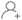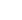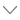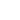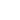# 加拿大纽芬兰纪念大学微积分课程预习秘诀!

## 1.什么是微分和积分?

a.微分：f'(a) = limh→0 [f(a+h) - f(h)]/h

b.积分：∫f(x) dx = f(x) + C

## 2.微分和积分规则

a.微分乘积法则：[f(x)g(x)]' = f'(x)g(x) + g'(x)f(x)

b.微分商法则：[f(x)/g(x)]' = [f'(x)g(x) - g'(x)f(x)]/[g(x)]2

c.微分链法则：[f(g(g(x))]' = f'(g(x)) × g'(x)

d.分式积分： ∫f(x)g(x) dx = f(x) ∫g(x) dx - ∫[f'(x) × ∫g(x) dx] dx

## 3.微分与积分的相似之处

a.它们都满足线性性质，即 d(f(x) ± g(x))/dx = d(f(x))/dx ± d(g(x))/dx 和 ∫[f(x) ± g(x)] dx = ∫f(x) dx ± ∫g(x)dx。

b.微分和积分互为逆过程。

c.它们满足标量乘法性质，即 d(kf(x))/dx = kd(f(x))/dx 和 ∫kf(x) dx = k ∫f(x)dx。

4.关于微分和积分的重要说明：### 相关老师推荐##### Niki英国##### Sofia新加坡### 热门问答

hmkt088